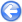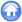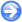### 6.7PASS: Wind-driven lake

Author
Stéphane Popinet
Command
gerris2D lake.gfs
Version
110325
Required files
lake.gfv
Running time
1 minutes 18 seconds

A simple test of a wind-driven lake with a 1/10 mesh stretching ratio. Due to the anisotropy of stretching, the hypre module needs to be used to solve the Poisson and diffusion problems efficiently. Further, with Neumann boundary conditions everywhere for the pressure, the resulting linear system is rank-deficient and needs to be "fixed" to avoid drift problems in HYPRE.

 Figure 94: Norm of the velocity and vectors for the stationary regime. The vertical scale is stretched by a factor of ten.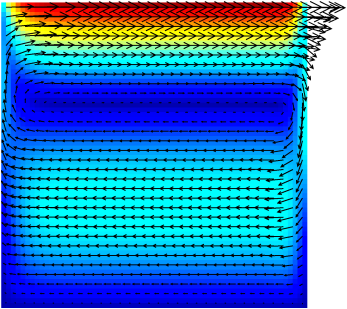#### 6.7.1PASS: Multi-layer Saint-Venant solver

Author
Stéphane Popinet
Command
sh river.sh
Version
120717
Required files
river.sh error.ref
Running time
21 seconds

A similar test but using the multi-layer hydrostatic Saint-Venant solver.

Figure 95 illustrates the velocity profile at the center of the lake and Figure 96 the rate of convergence with an increasing number of layers.

 Figure 95: Numerical and analytical velocity profiles at the center of the lake.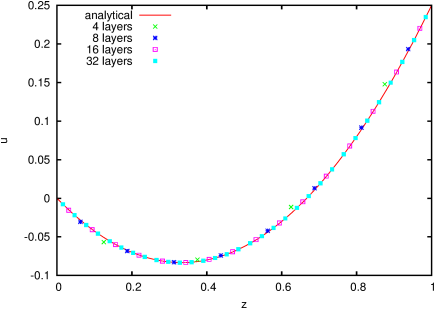Figure 96: Convergence of the error between the numerical and analytical solution with the number of layers.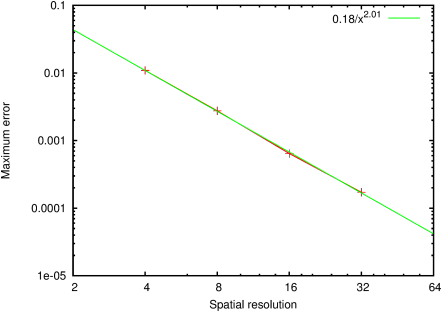#### 6.7.2PASS: Wind-driven stratified lake

Author
Stéphane Popinet
Command
sh stratified.sh
Version
121116
Required files
stratified.sh error.ref field.awk thermo.awk
Running time
1 minutes 46 seconds

We now consider the case of a stratified lake. To model the stable thermal stratification often observed in lakes, we assume a vertical density profile of the form

 ρ (z) = ρ0 − Heaviside (z − H + Hthermocline) Δ ρ .

If Δ ρ / ρ0 is small compared to one, we can use a Boussinesq approximation and this results in an additional source term − gHeaviside (zH + Hthermocline) for the vertical component of velocity, with g′ the reduced gravity

g′ =
 Δ ρ ρ0
g

Through dimensional arguments one can find that the equilibrium slope αt of the thermocline should verify the relation

 g′ αt ∝ ν τ,

for vanishing αt.

We then need to consider two additional independent dimensionless parameters

• b = 1 − Hthermocline / H, set to 3 / 4 in what follows,
• and the thermocline slope
αt =  ν τ g′ H

If we assume parallel flow, an analytical solution can be sought for the horizontal velocity profile in a vertical cross-section, of the form

 u (z) = A1 z2 + B1 z + C1 for z > b, u (z) = A2 z2 + B2 z + C2 for z ≤ b

Using boundary and volume conservation conditions one easily finds (for b = 3 / 4)

u (z) =
 30 z2 − 47 z + 18 13
for z > 3 / 4,
u (z) =
 − 2 z2 + z 13
for z ≤ 3 / 4.

This can be used to refine the estimates of the thermocline and surface slopes. Assuming hydrostatic balance, the horizontal pressure gradient is given by

 ∂x p = g α′ for z > 3 / 4 + αt′ x, ∂x p = g α′ + g′ αt′ for z ≤ 3 / 4 + αt′ x.

Balancing the horizontal pressure gradients and viscous stress then gives

α′=
 60 13
α
αt=
−
 64 13
αt

The (pseudo) steady-state density perturbation is illustrated in Figure 97 and the corresponding free-surface and thermocline slopes in Figure 100.

Figure 98 illustrates the velocity profile at the center of the lake and Figure 99 the rate of convergence with an increasing number of layers.

 Figure 97: Density perturbation Δρ for 64 layers. Numerical diffusion is most noticeable in the upwelling on the left-hand-side.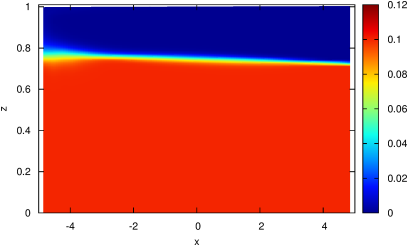Figure 98: Numerical and analytical velocity profiles at the center of the lake.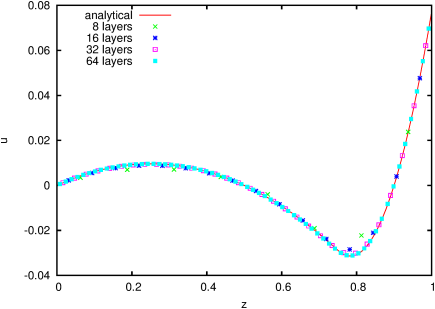Figure 99: Convergence of the error between the numerical and analytical solution with the number of layers.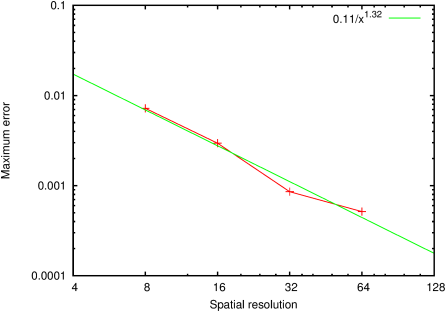Figure 100: Profiles of (a) the free surface and (b) the thermocline compared with the analytical solutions.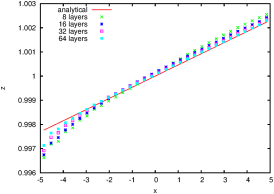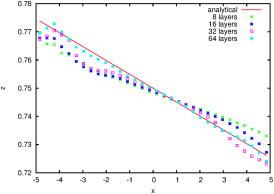(a) (b)